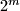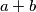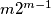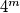### IMO Shortlist 2014 problem C2

Kvaliteta:
Avg: 3,0
Težina:
Avg: 6,0

We have$2^m$ sheets of paper, with the number$1$ written on each of them. We perform the following operation. In every step we choose two distinct sheets; if the numbers on the two sheets are$a$ and$b$, then we erase these numbers and write the number$a + b$ on both sheets. Prove that after$m 2^{m-1}$ steps, the sum of the numbers on all the sheets is at least$4^m$.

(Iran)

Izvor: https://www.imo-official.org/problems/IMO2014SL.pdf